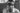## How to use LINQ in PowerShell to compare arrays

LINQ stands for Language Integrated Query and if you use LINQ in PowerShell it might boost the performance of your scripts. In this article, I’ll show you how to use LINQ in PowerShell for comparing arrays.

In this article, I will show you except and intersect of LINQ. There are many more methods. If you are interested in other methods, check out the official documentation of Microsoft.

## Concept of using LINQ in PowerShell

We have three amounts red, orange and yellow. The aim is to only get a specific amount e.g. only the red portion.

```\$Red = @( "A", "B", "C")
\$Yellow = @("C","D","E")```

We also can do this for integer values.

```\$Red = @( 1..5)
\$Yellow = @(4..10)```

## Using LINQ for Strings in arrays

I will show you how to use LINQ for string in this chapter. We will cover the methods except and intersect.

### String – Get the red amount

In order to get the red amount, you have to do following:

```\$Red = @( "A", "B", "C")
\$Yellow = @("C","D","E")

\$Left  = [string[]]\$Red
\$Right = [string[]]\$Yellow

[string[]][Linq.Enumerable]::Except(\$Left, \$Right)```

By doing this, you’ll get only A and B:

### String – Get the yellow amount

In order to get the red amount, you have to change Right and Left in the LINQ cmdlet:

```\$Red = @( "A", "B", "C")
\$Yellow = @("C","D","E")

\$Left  = [string[]]\$Red
\$Right = [string[]]\$Yellow

[string[]][Linq.Enumerable]::Except(\$Right, \$Left)```

By doing this, you’ll get only D and E:

### String – Get the orange amount

If we want to get the orange amount, we have to make use of intersect

```\$Red = @( "A", "B", "C")
\$Yellow = @("C","D","E")

\$Left  = [string[]]\$Red
\$Right = [string[]]\$Yellow

[string[]][Linq.Enumerable]::Intersect(\$Left, \$Right)```

### String – Get everything except orange

```\$Red = @( "A", "B", "C")
\$Yellow = @("C","D","E")

\$Left  = [string[]]\$Red
\$Right = [string[]]\$Yellow

[string[]]([Linq.Enumerable]::Except(\$Left, \$Right) + [Linq.Enumerable]::Except(\$Right, \$Left))```

By doing this, you’ll everything but not C:

## Using LINQ for integers in arrays

I will show you how to use LINQ for integers in this chapter. We will cover the methods except and intersect.

### Integer – Get the red amount

In order to get the red amount, you have to do following:

```\$Red = @( 1..5)
\$Yellow = @(4..10)

\$Left  = [int[]]\$Red
\$Right = [int[]]\$Yellow

[int[]][Linq.Enumerable]::Except(\$Left, \$Right)```

By doing this, you’ll get only 1, 2, 3:

### Integer – Get the yellow amount

In order to get the red amount, you have to change Right and Left in the LINQ cmdlet:

```\$Red = @( 1..5)
\$Yellow = @(4..10)

\$Left  = [int[]]\$Red
\$Right = [int[]]\$Yellow

[int[]][Linq.Enumerable]::Except(\$Right, \$Left)```

By doing this, you’ll get only 6, 7, 8, 9, 10:

### Integer – Get the orange amount

If we want to get the orange amount, we have to make use of intersect

```\$Red = @( 1..5)
\$Yellow = @(4..10)

\$Left  = [int[]]\$Red
\$Right = [int[]]\$Yellow

[int[]][Linq.Enumerable]::Intersect(\$Right, \$Left)```

### Integer – Get everything except orange

```\$Red = @( 1..5)
\$Yellow = @(4..10)

\$Left  = [int[]]\$Red
\$Right = [int[]]\$Yellow

[int[]]([Linq.Enumerable]::Except(\$Left, \$Right) + [Linq.Enumerable]::Except(\$Right, \$Left))```

By doing this, you’ll everything but not 5:

You might be interested in getting items faster interactively. This article might help:

Get Items / Files interactively – SPO Scripts

Image by Tumisu from Pixabay